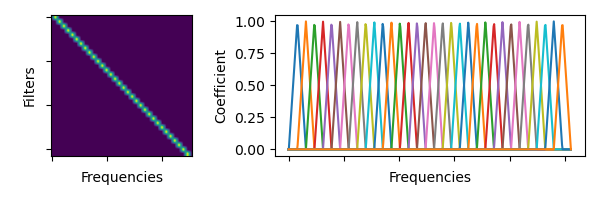Shortcuts

# torchaudio.functional.linear_fbanks¶

torchaudio.functional.linear_fbanks(n_freqs: int, f_min: float, f_max: float, n_filter: int, sample_rate: int) [source]

Creates a linear triangular filterbank.Note

For the sake of the numerical compatibility with librosa, not all the coefficients in the resulting filter bank has magnitude of 1.Parameters:
• n_freqs (int) – Number of frequencies to highlight/apply

• f_min (float) – Minimum frequency (Hz)

• f_max (float) – Maximum frequency (Hz)

• n_filter (int) – Number of (linear) triangular filter

• sample_rate (int) – Sample rate of the audio waveform

Returns:

Triangular filter banks (fb matrix) of size (n_freqs, n_filter) meaning number of frequencies to highlight/apply to x the number of filterbanks. Each column is a filterbank so that assuming there is a matrix A of size (…, n_freqs), the applied result would be A * linear_fbanks(A.size(-1), ...).

Return type:

Tensor

## Docs

Access comprehensive developer documentation for PyTorch

View Docs

## Tutorials

Get in-depth tutorials for beginners and advanced developers

View Tutorials